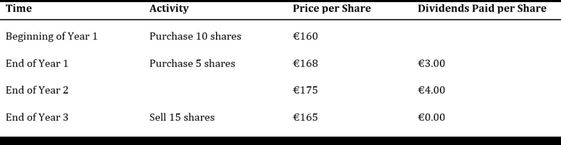# TWR questionHow would you find the TWR in this question?

would it be CF = -1600 CF1= (-840+30) CF2 = 60 CF3= 2625 ?

Use the cash flow buttons when you’re looking for the money-weighted return.

For the time-weighted return, calculate each year’s holding period return, then compound them.

Got it. But is the CF function correct for MWR?

Absitively!

1 Like